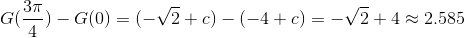# AP Calculus BC : Volume of Cross Sections and Area of Region

## Example Questions

2 Next →

### Example Question #11 : Volume Of Cross Sections And Area Of Region

Find the area bound by the curve of g(t), the x and y axes, and the line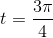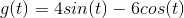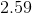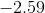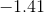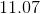Explanation:

Find the area bound by the curve of g(t), the x and y axes, and the lineWe are asked to find the area under a curve. This sounds like a job for an integral.

We need to integrate our function from 0 to. These will be our limits of integration. With that in mind, our integral look like this.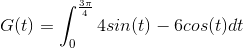Now, we need to recall the rules for integrating sine and cosine.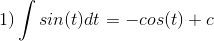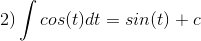With these rules in mind, we can integrate our original function to get: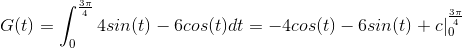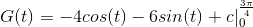Now, we just need to evaluate our new function at the given limits. Let's start with 0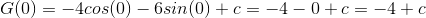And our upper limit...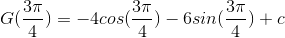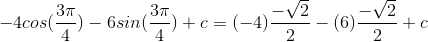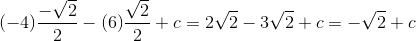Now, we need to take the difference between our limits: